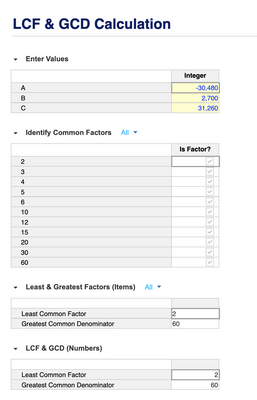# How to find GCD of 3 numbers?Hello Community,

I have a condition, where I have to calculate the "Greatest Common Divisor(GCD/GCF)" of three numbers in Anaplan so that I can utilize that GCD value to find the ratio.
In excel we have GCD function and directly we obtain the GCD value. So, is there any way we can do that in Anaplan.

Thanks

Puneeth HP

Success is the Intersection of Dreams and Hardwork!

Tagged:

•Use the modulus function MOD(x,y) to find common factors among a very big numbered list of factors. Use summary First non-blank or Last non-blank to find the least common factor and the greatest common denominator, respectively.

List 1: Name “Inputs” Three items “A”, “B”, “C” and with a top level “All”.

List 2: Name “Factors#”, numbered list with like 9,999 items, add a top level item: "All"
Code = 2,3,…10,000   <<  Skip 1 because it is a factor of every integer. The LCF must be > 1.
Property (text) Display Name = CODE(ITEM(Factors#))
Property (number) NumVal = VALUE(Display Name)

Module:
Name: “Enter Values”
Applies To: Inputs
Line Item: “Integer”
format: number, decimals 0
formula: none

Module:
name “Identify Common Factors”
Applies To: Values, Factors#
Line Item:
Name: “Is Factor?”,
format: Boolean,
formula = MOD(Enter Values.Integer, Factors#.NumVal) = 0
Summary: All

Module:
Name: “Least & Greatest Factors (Items)”
Applies To: Factors#
Line Item: “Least Common Factor”
Format: List, Factors#
Formula: IF Identify Common Factors.Is Factor? THEN ITEM(Factors#) ELSE BLANK
Summary: First non-blank.   <<< this puts the least common factor item in the top level item Factors#.All.
Line Item: “Greatest Common Denominator”
Format: List, Factors#
Formula: IF Identify Common Factors.Is Factor? THEN ITEM(Factors#) ELSE BLANK
Summary: Last non-blank.   <<< this puts the greatest common denominator item in the top level item Factors#.All.

Module:
Name: "LCF & GCD (Numbers)"
Applies To: <none>
Line Item:
Name: Least Common Factor
Format: Number, Decimals 0
Formula:  VALUE(CODE('Least & Greatest Factors (Items)'.Least Common Factor))
Line Item:
Name: Greatest Common Denominator
Format: Number, Decimals 0
Formula:  VALUE(CODE('Least & Greatest Factors (Items)'.Greatest Common Denominator))•Unfortunately, there is no GCD function in Anaplan (you can suggest this an Idea). You could theoretically try to rebuild the Euclidean algorithm for calculating GCD, but this would require a large number of line items and likely not the most efficient way to solve the problem. Depending on how often this calculation needs to be performed and the data involved, I would recommend looking at ways to calculate this outside of Anaplan and load in the result.

I am also curious about the use case. Is there any particular reason you need to express the ratios in whole numbers, or could you express your ratios relative to 1? e.g. 3:1, 7.5:1, 46.77765:1, etc.

•@hendersonmj
Thanks for this resolution.
Just one thought, what if GCD > 9999.

Thanks,
Puneeth HP

Success is the Intersection of Dreams and Hardwork!